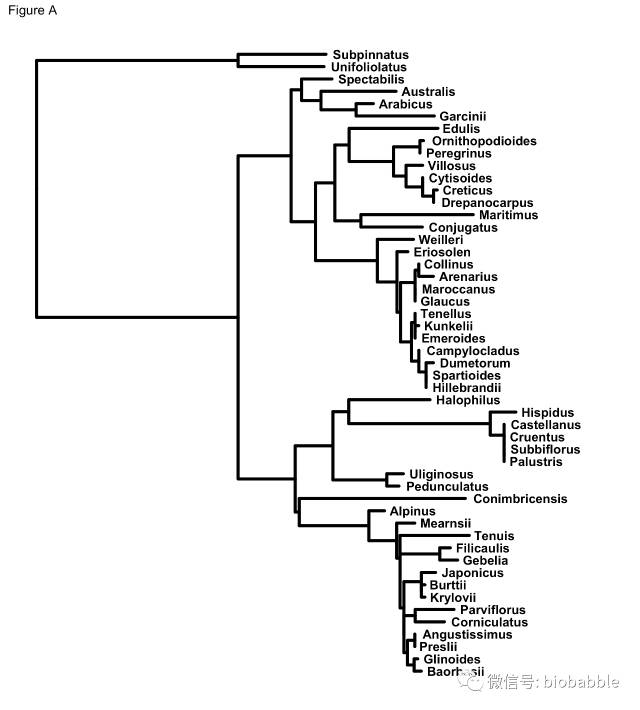# bootstrap分段标记

2017年5月4日 137点热度 0人点赞 0条评论

``tree <- read.newick("RMI.phy_phyml_tree_rooted_labeled.txt", node.label='support') root <- getRoot(tree@phylo)  p <- ggtree(tree, color="black", size=1.5, linetype=1,  ladderize=TRUE) + ggtitle(label="Figure A") + geom_tiplab(size=4.5, hjust = -0.060, fontface="bold") +  xlim(0, 0.09)````p <- p + geom_point2(aes(subset=!isTip & node != root, fill=cut(support, c(0, 700, 900, 1000))), shape=21, size=4) + theme_tree(legend.position=c(0.2, 0.3))````p + scale_fill_manual(values=c("white", "grey", "black"), guide='legend', name='Bootstrap Percentage(BP)', breaks=c('(900,1e+03]', '(700,900]', '(0,700]'), labels=expression(BP>=90,70 <= BP * " < 90", BP < 70))``51920bootstrap分段标记